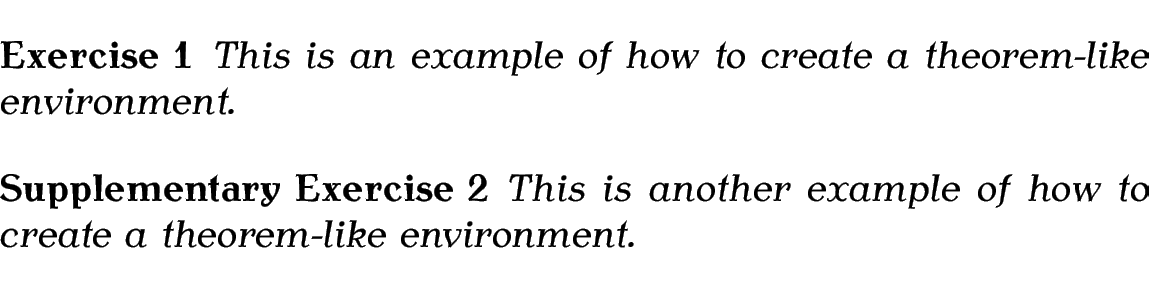# 4.7 Theorems

A PhD thesis can often contain theorems, lemmas, definitions etc. The LaTeX kernel comes with the command:

\newtheorem{<name>}[<counter>]{<title>}[<outer counter>]

which can be used to create an environment called <name> that has an optional argument. Each instance of the environment starts with <title> followed by the associated counter value. If <counter> is present, the new environment uses that counter instead of having a new counter defined for it. If <outer counter> is present, the environment counter is reset every time <outer counter> is incremented. The optional arguments are mutually exclusive.

In the example below, I've use \newtheorem to define a new environment called theorem, which has an associated counter, also called theorem, that is dependant on the chapter counter.

% in the preamble:
\newtheorem{theorem}{Theorem}[chapter]

% later in the document:
\begin{theorem}
If proposition $P$ is a tautology
then $\sim P$ is a contradiction,
and conversely.
\end{theorem}

Resulting output:The optional argument to the new environment can be used to add a caption. Modifying the above example (changes shown like this):

% in the preamble:
\newtheorem{theorem}{Theorem}[chapter]

% later in the document:
\begin{theorem}[Tautologies and Contradictions]
If proposition $P$ is a tautology
then $\sim P$ is a contradiction,
and conversely.
\end{theorem}

Resulting output:Here's an example that uses the first optional argument of \newtheorem:

% in the preamble:
\newtheorem{exercise}{Exercise}
\newtheorem{suppexercise}[exercise]{Supplementary Exercise}

% later in the document:
\begin{exercise}
This is an example of how to create a theorem-like environment.
\end{exercise}
\begin{suppexercise}
This is another example of how to create a theorem-like environment.
\end{suppexercise}

Result:Unfortunately there isn't a great deal of flexibility with the environment appearance. [Theorem bodies printed in a roman font]However there are various packages available that provide enhancements to this basic command, allowing you to adjust the appearance to suit your requirements. There seem to be two main contenders: amsthm and ntheorem. Both have advantages and disadvantages. For example, ntheorem is more flexible but amsthm is more robust. Therefore I'm going to describe both, and you will have to decide which one you prefer.

Note:

If you are using either packages with amsmath, you must load amsmath first:

or

With both amsthm and ntheorem, you can still define new theorem-like environments using \newtheorem, but there is also a starred version of that command, which can be used to define unnumbered theorem-like environments.

Example:

Suppose I want to have an unnumbered remark environment, I can define the environment like this:

% in the preamble:
\newtheorem*{note}{Note}

% later in the document:
\begin{note}
This is a note about something.
\end{note}

Result:This book is also available as A4 PDF or 12.8cm x 9.6cm PDF or paperback (ISBN 978-1-909440-02-9).

© 2013 Dickimaw Books. "Dickimaw", "Dickimaw Books" and the Dickimaw parrot logo are trademarks. The Dickimaw parrot was painted by Magdalene Pritchett.